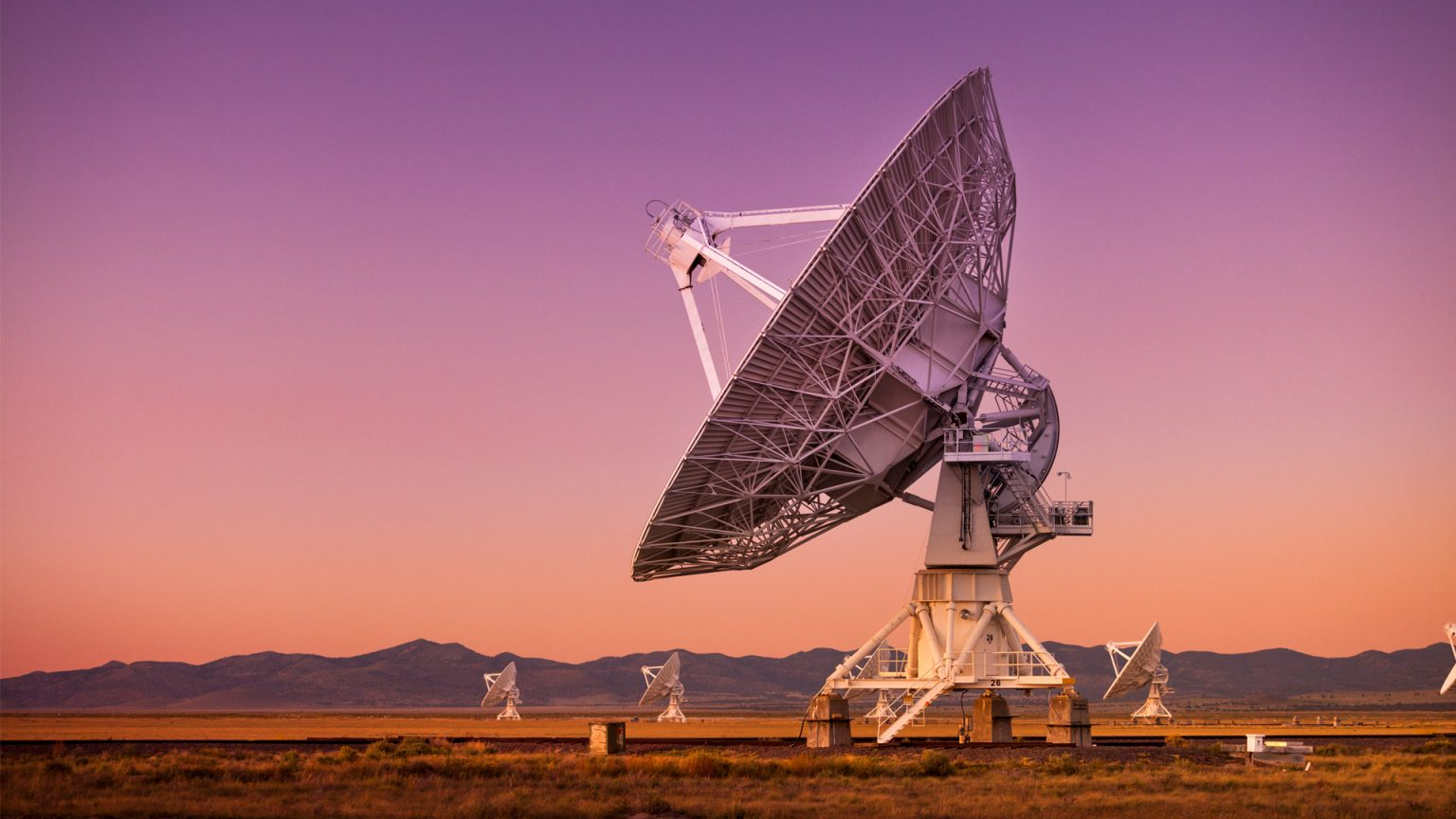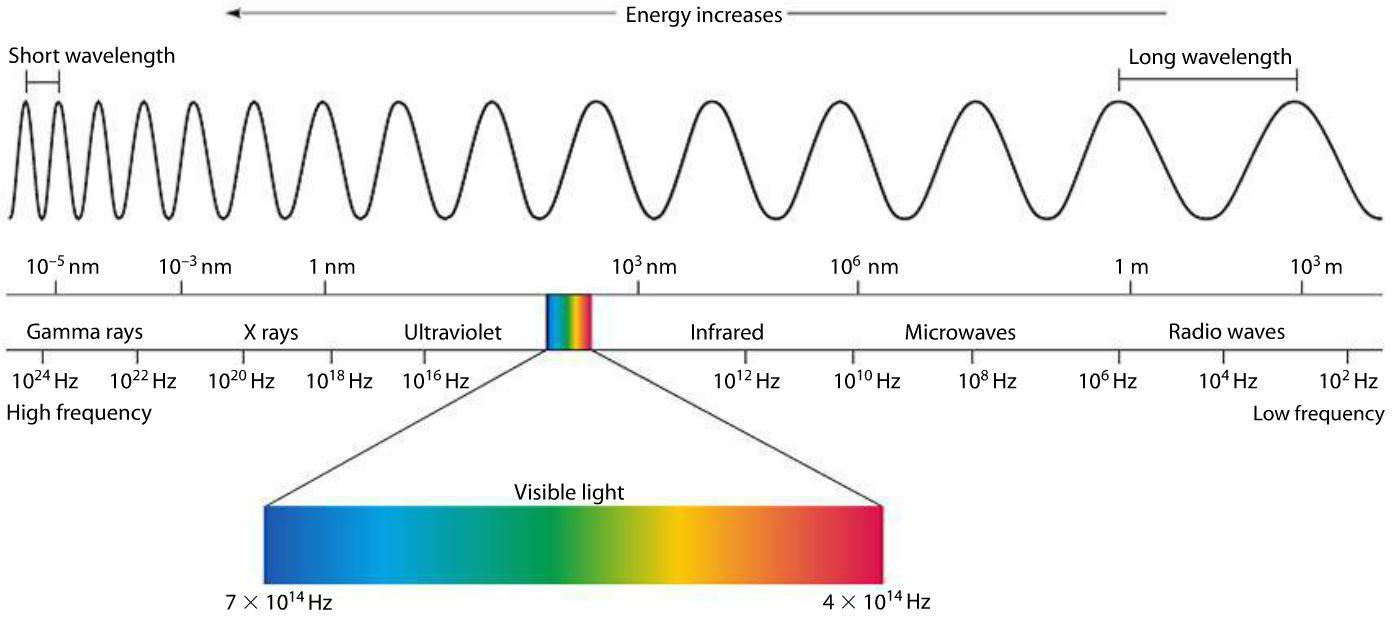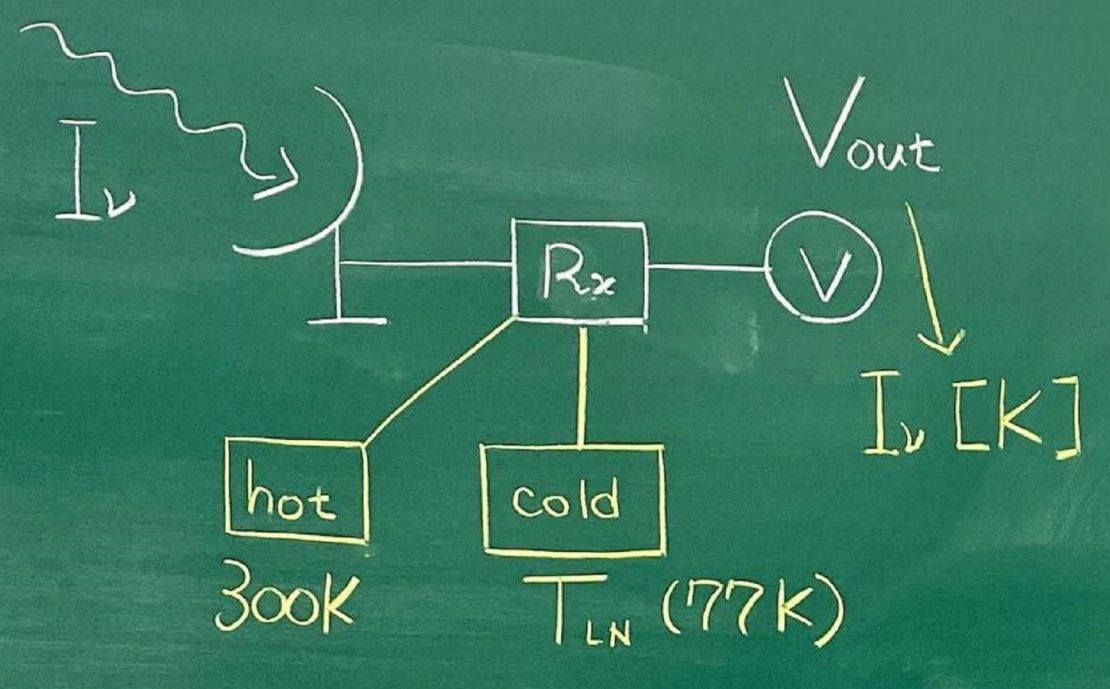## 黑体辐射与亮温度(Brightness Temperature)的定义

我们知道，物体的热辐射谱是黑体谱，其谱仅用一个参数便可以去描述，那就是温度。黑体辐射的强度随频率$\nu$的变化可以用下式表示：

$B_\nu(\nu,T)=\frac{2h\nu^3}{c^2}\frac{1}{exp(\frac{h\nu}{kT})-1}~.$

根据爱因斯坦的光电理论，对于电磁波而言，若把其看成粒子，那么其能量为$h\nu$。如果光子的频率越低，相对应波长$\lambda$越长，其能量就越低。那么对于射电频段，从下图可以看出，其对应的是波长较长的电磁波：所以，对于射电波而言($h\nu \ll kT$)，黑体辐射$B_\nu$便可以近似为如下形式：

$B_\nu \approx \frac{2kT\nu^2}{c^2}~,$

$T\approx \frac{B_\nu c^2}{2k\nu}~.$

所以，重点来了，假设处在射电频段的发射某信号的源的辐射机制是黑体辐射，那么通过Rayleigh-Jeans law关系计算得到的温度，便称作亮温度, Brightness Temperature$T_b$. ⚠️请注意，由于我们这里是假设源是黑体辐射，所以只有当其真正是黑体辐射的时候，这个温度$T_b$才代表真正的物理温度，否则这个温度仅仅是一个与$I_\nu$的等价量罢了，只不过是换了个单位。

## 那么，为啥要用温度来描述信号呢？

在上面笔者介绍了黑体辐射以及亮温度的定义，现在可以开始回答开篇的问题了：为啥不直接用$I_\nu$来描述信号，而非要整个温度呢？难道是射电工作者比较有个性，要搞特殊特立独行😎？哈哈哈答案自然不是，其原因主要是因为在射电望远镜频段用于校准的时候，为了方便，通常人们会使用温度这个概念，且听我缓缓道来😁
我们在天文领域使用的射电望远镜，如下图所示(图片来自Nakashima黑板手绘图)实际上工作的时候的流程是：接收到电磁波信号->将电磁波信号转换为电信号->控制台记录📝.那么相信看到这里，你已经知晓了为什么在射电天文领域，大家要用这么一个“模棱两可”的温度的概念来描述信号了吧。逻辑如下：

以上，便是整套逻辑了，希望能够解答你的困惑😄